The economic value added (EVA) is a measure of the dollar surplus value created by an investment or a portfolio of investments. It is computed as the product of the "excess return" made on an investment or investments and the capital invested in that investment or investments.

Economic Value Added = (Return on Capital Invested – Cost of Capital) (Capital Invested) = After tax operating income – (Cost of Capital) (Capital Invested)

In this section, we will begin by looking at the measurement of economic value added, then consider its links to discounted cash flow valuation and close with a discussion of its limitations as a value enhancement tool.

## Calculating EVA

The definition of EVA outlines three basic inputs we need for its computation - the return on capital earned on investments, the cost of capital for those investments and the capital invested in them. In measuring each of these, we will make many of the same adjustments we discussed in the context of discounted cash flow valuation.

How much capital is invested in existing assets? One obvious answer is to use the market value of the firm, but market value includes capital invested not just in assets in place but in expected future growth. Since we want to evaluate the quality of assets in place, we need a measure of the market value of just these assets. Given the difficulty of estimating market value of assets in place, it is not surprising that we turn to the book value of capital as a proxy for the market value of capital invested in assets in place. The book value, however, is a number that reflects not just the accounting choices made in the current period, but also accounting decisions made over time on how to depreciate assets, value inventory and deal with acquisitions. At the minimum, the three adjustments we made to capital invested in the discounted cashflow valuation – converting operating leases into debt, capitalizing R&D expenses and eliminating the effect of one-time or cosmetic charges – have to be made when computing EVA as well. The older the firm, the more extensive the adjustments that have to be made to book value of capital to get to a reasonable estimate of the market value of capital invested in assets in place. Since this requires that we know and take into account every accounting decision over time, there are cases where the book value of capital is too flawed to be fixable. Here, it is best to estimate the capital invested from the ground up, starting with the assets owned by the firm, estimating the market value of these assets and cumulating this market value.

To evaluate the return on this invested capital, we need an estimate of the after-tax operating income earned by a firm on these investments. Again, the accounting measure of operating income has to be adjusted for operating leases, R&D expenses and one-time charges to compute the return on capital.

The third and final component needed to estimate the economic value added is the cost of capital. In keeping with our arguments both in the investment analysis and the discounted cash flow valuation sections, the cost of capital should be estimated based upon the market values of debt and equity in the firm, rather than book values. There is no contradiction between using book value for purposes of estimating capital invested and using market value for estimating cost of capital, since a firm has to earn more than its market value cost of capital to generate value. From a practical standpoint, using the book value cost of capital will tend to understate cost of capital for most firms and will understate it more for more highly levered firms than for lightly levered firms. Understating the cost of capital will lead to overstating the economic value added.

EVA Computation in Practice

During the 1990s, EVA was promoted most heavily by Stern Stewart, a New York based consulting firm. The firm’s founders Joel Stern and Bennett Stewart became the foremost evangelists for the measure. Their success spawned a whole host of imitators from other consulting firms, all of which were variants on the excess return measure.

Stern Stewart, in the process of applying this measure to real firms found that it had to modify accounting measures of earnings and capital to get more realistic estimates of surplus value. Bennett Stewart, in his book titled “The Quest for Value” mentions some of the adjustments that should be made to capital invested including adjusting for goodwill (recorded and unrecorded). He also suggests adjustments that need to be made to operating income including the conversion of operating leases into financial expenses.

Many firms that adopted EVA during this period also based management compensation upon measured EVA. Consequently, how it was defined and measured became a matter of significant concern to managers at every level.

## Economic Value Added, Net Present Value and Discounted Cashflow Valuation

One of the foundations of investment analysis in traditional corporate finance is the net present value rule. The net present value (NPV) of a project, which reflects the present value of expected cash flows on a project, netted against any investment needs, is a measure of dollar surplus value on the project. Thus, investing in projects with positive net present value will increase the value of the firm, while investing in projects with negative net present value will reduce value. Economic value added is a simple extension of the net present value rule. The net present value of the project is the present value of the economic value added by that project over its life.where EVAt is the economic value added by the project in year t and the project has a life of n years.

This connection between economic value added and NPV allows us to link the value of a firm to the economic value added by that firm. To see this, let us begin with a simple formulation of firm value in terms of the value of assets in place and expected future growth.

Firm Value = Value of Assets in Place + Value of Expected Future Growth

Note that in a discounted cash flow model, the values of both assets in place and expected future growth can be written in terms of the net present value created by each component.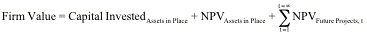Substituting the economic value added version of net present value into this equation, we get: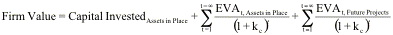Thus, the value of a firm can be written as the sum of three components, the capital invested in assets in place, the present value of the economic value added by these assets and the expected present value of the economic value that will be added by future investments.

###### Illustration 32.1: Discounted Cashflow Value and Economic Value Added

Consider a firm that has existing assets in which it has capital invested of \$100 million. Assume these additional facts about the firm.

1.     The after-tax operating income on assets in place is \$15 million. This return on capital of 15% is expected to be sustained in the future and the company has a cost of capital of 10%.

2.     At the beginning of each of the next 5 years, the firm is expected to make investments of \$10 million each. These investments are also expected to earn 15% as a return on capital and the cost of capital is expected to remain 10%.

3.     After year 5, the company will continue to make investments and earnings will grow 5% a year, but the new investments will have a return on capital of only 10%, which is also the cost of capital.

4.     All assets and investments are expected to have infinite lives. Thus, the assets in place and the investments made in the first five years will make 15% a year in perpetuity, with no growth.

This firm can be valued using an economic value added approach, as shown in Table 32.1.

Table 32.1: Economic Value Added Valuation of Firm

 Capital Invested in Assets in Place \$100 + EVA from Assets in Place\$ 50 + PV of EVA from New Investments in Year 1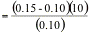\$ 5 + PV of EVA from New Investments in Year 2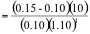\$ 4.55 + PV of EVA from New Investments in Year 3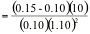\$ 4.13 + PV of EVA from New Investments in Year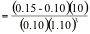\$ 3.76 + PV of EVA from New Investments in Year 5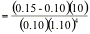\$ 3.42 Value of Firm \$ 170.85

Note that the present values are computed assuming that the cash flows on investments are perpetuities. In addition, the present value of the economic value added by the investments made in future years are discounted to the present, using the cost of capital. To illustrate, the present value of the economic value added by investments made at the beginning of year 2 is discounted back two years. The value of the firm, which is \$170.85 million, can be written using the firm value equation.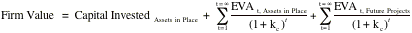\$170.85 mil=    \$100 mil                            + \$50 mil                     +  \$20.85 mil

The value of existing assets is therefore \$150 million and the value of future growth opportunities is \$ 20.85 million.

Another way of presenting these results is in terms of Market Value Added (MVA). The market value added, in this case, is the difference between the firm value of \$170.85 million and the capital invested of \$100 million, which yields \$70.85 million. This value will be positive only if the return on capital is greater than the cost of capital and will be an increasing function of the spread between the two numbers. Conversely, the number will be negative if the return on capital is less than the cost of capital.

Note that although the firm continues to grow operating income and makes new investments after the fifth year, these marginal investments create no additional value because they earn the cost of capital. A direct implication is that it is not growth that creates value, but growth in conjunction with excess returns. This provides a new perspective on the quality of growth. A firm can be increasing its operating income at a healthy rate, but if it is doing so by investing large amounts at or below the cost of capital, it will not be creating value and may actually be destroying it.

This firm could also have been valued using a discounted cash flow valuation, with free cashflows to the firm discounted at the cost of capital. Table 32.2 shows expected free cash flows and the firm value, using the cost of capital of 10% as the discount rate. In looking at this valuation, note the following.

·      The capital expenditures occur at the beginning of each year and thus are shown in the previous year. The investment of \$10 million in year 1 is shown in year 0, the year 2 investment in year 1 and so on.

·      In year 5, the net investment needed to sustain growth is computed by using two assumptions – that growth in operating income would be 5% a year beyond year 5, and that the return on capital on new investments starting in year 6 (which is shown in year 5) would be 10%.

Net Investment5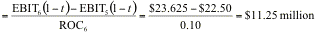The value of the firm obtained by discounting free cash flows to the firm at the cost of capital is \$170.85, which is identical to the value obtained using the economic value added approach.

Table 32.2: Firm Value using DCF Valuation

 0 1 2 3 4 5 Term. Year EBIT (1-t) from Assets in Place \$0.00 \$        15.00 \$        15.00 \$        15.00 \$        15.00 \$        15.00 EBIT(1-t) from Investments - Yr 1 \$           1.50 \$           1.50 \$           1.50 \$           1.50 \$           1.50 EBIT(1-t) from Investments - Yr 2 \$           1.50 \$           1.50 \$           1.50 \$           1.50 EBIT(1-t) from Investments - Yr 3 \$           1.50 \$           1.50 \$           1.50 EBIT(1-t) from Investments - Yr 4 \$           1.50 \$           1.50 EBIT(1-t) from Investments - Yr 5 \$           1.50 Total EBIT(1-t) \$        16.50 \$        18.00 \$        19.50 \$        21.00 \$        22.50 \$        23.63 - Net Capital Expenditures \$10.00 \$        10.00 \$        10.00 \$        10.00 \$        10.00 \$        11.25 \$        11.81 FCFF (\$10) \$           6.50 \$           8.00 \$           9.50 \$        11.00 \$        11.25 \$        11.81 PV of FCFF (\$10) \$           5.91 \$           6.61 \$           7.14 \$           7.51 \$           6.99 Terminal Value \$      236.25 PV of Terminal Value \$      146.69 Value of Firm \$170.85 Return on Capital 15% 15% 15% 15% 15% 15% 10% Cost of Capital 10% 10% 10% 10% 10% 10% 10%

 As an illustration, computing the return on capital at Microsoft using the market value of the firm, instead of book value, results in a return on capital of about 3%. It would be a mistake to view this as a sign of poor investments on the part of the firm's managers.

 This is true, though, only if the expected present value of the cash flows from depreciation is assumed to be equal to the present value of the return of the capital invested in the project. A proof of this equality can be found in my paper on value enhancement in the Contemporary Finance Digest in 1999.

 Note that this assumption is purely for convenience, since it makes the net present value easier to compute.